Monday 18th January 2021

CBSE Guess > Papers > Question Papers > Class XII > 2003 > Mathematics > Compartment Outside Delhi Set -II

MATHEMATICS—2003 (Set II—Compartment Outside Delhi)

Note: Except for the following questions, all the remaining question have been asked in Set I .

SECTION - A

Q. 2. Find the values offor which the following holds: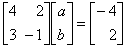Q. 4. Evaluate:Q. 7. Using properties of determinants, show that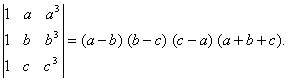Q. 12. Evaluate: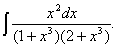Q. 13. The two lines of regression are given by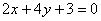andFindand the co-efficient of correlation between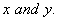Q. 14. A particle moves along the curve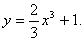Find the points on the curve at which the y-coordinate is changing twice as first as x-coordinate.

Q. 18. Using integration, find the area of the triangle ABC whose vertices are A(3, 0), B(4, 5) and C(5, 1).

SECTION - B

Q. 21. Find the values ofso that the vectors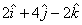andare (i) parallel, (ii) perpendicular to each other.

Q. 22. Find the equation of the plane passing through the line of intersection of the planesand parallel to the line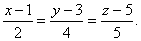SECTION - C

Q. 21. For a near normal distribution, Karl Pearson's co-efficient of skewness is 0.6 the standard deviation of the distribution is 13 and the mean is 42. Find the median and mode.

Q. 22. The first three moments about the value 4 of a variable are -1.5, 17 and -30 respectively. Find the mean, variance and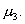Mathematics 2003 Question Papers Class XII Delhi Outside Delhi Compartment Delhi Compartment Outside DelhiSet ISet ISet ISet ISet IISet IISet IISet IISet IIISet III

CBSE 2003 Question Papers Class XII